Une extension de l'ensemble de Mandelbrot en 3D et en OpenGL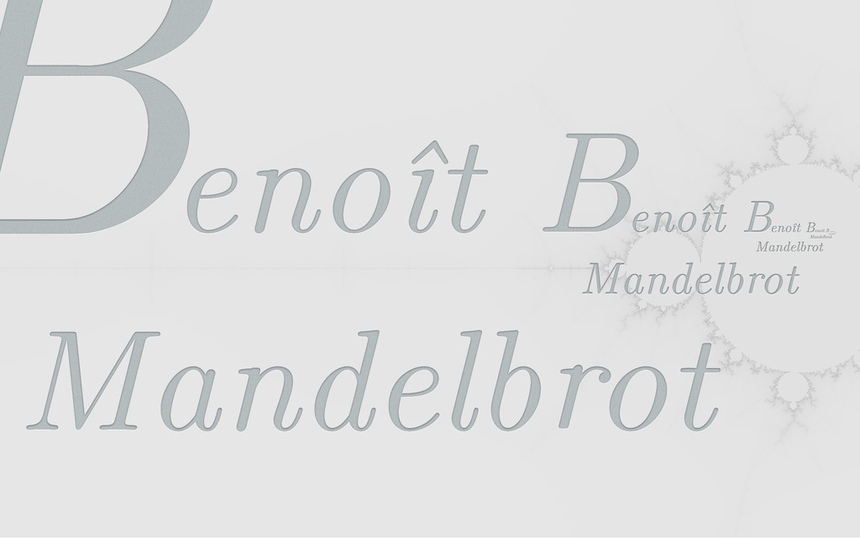tlpl: Un exemple progressif d’utilisation d’Haskell. Vous pourrez voir un ensemble de Mandelbrot étendu à la troisième dimension. De plus le code sera très propre. Les détails de rendu sont séparés dans un module externe. Le code descriptif intéressant est concentré dans un environnement pur et fonctionnel. Vous pouvez vous inspirer de ce code utilisant le paradigme fonctional dans tous les languages.

Introduction

In my preceding article I introduced Haskell.

As Haskell is designed for functional paradigm, it is easier to use in this context. In reality, the firsts sections will use an imperative paradigm. As you can use functional paradigm in imperative language, you can also use imperative paradigm in functional languages.

This article is about creating an useful and clean program. It can interact with the user in real time. It uses OpenGL, a library with imperative programming foundations. Despite this fact, most of the final code will remain in the pure part (no IO).

• Haskell programmer looking for an OpengGL tutorial.
• People interested in program organization (programming language agnostic).
• Fractal lovers and in particular 3D fractal.
• People interested in user interaction in a functional paradigm.

I had in mind for some time now to make a Mandelbrot set explorer. I had already written a command line Mandelbrot set generator in Haskell. This utility is highly parallel; it uses the repa package1.

This time, we will not parallelize the computation. Instead, we will display the Mandelbrot set extended in 3D using OpenGL and Haskell. You will be able to move it using your keyboard. This object is a Mandelbrot set in the plan (z=0), and something nice to see in 3D.

Here are some screenshots of the result:

And you can see the intermediate steps to reach this goal: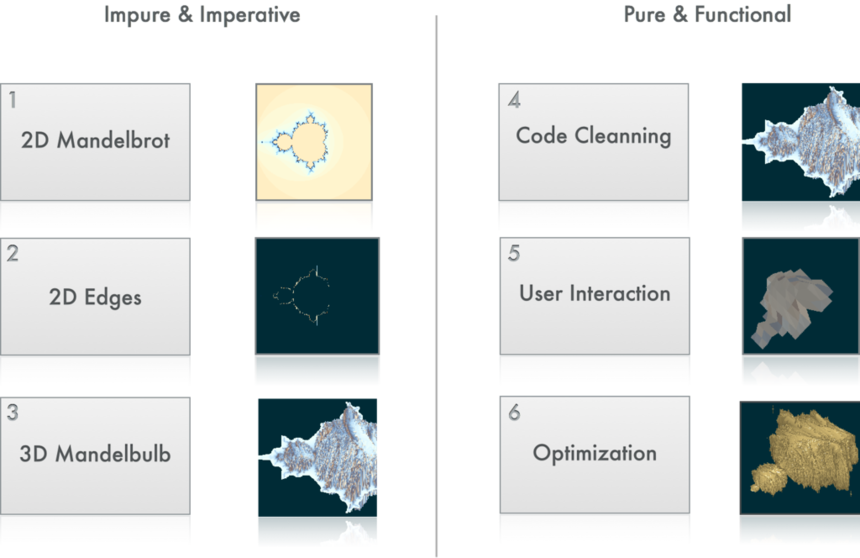From the 2nd section to the 4th it will be dirtier and dirtier. We start cleaning the code at the 5th section.

First version

We can consider two parts. The first being mostly some boilerplate2. And the second part more focused on OpenGL and content.

Let’s play the song of our people

For efficiency reason3, I will not use the default Haskell Complex data type.

We declare some useful functions for manipulating complex numbers:

Let us start

We start by giving the main architecture of our program:

Mainly, we initialize our OpenGL application. We declared that the function display will be used to render the graphics:

Also here, there is only one interesting line; the draw will occur in the function drawMandelbrot.

This function will provide a list of draw actions. Remember that OpenGL is imperative by design. Then, one of the consequence is you must write the actions in the right order. No easy parallel drawing here. Here is the function which will render something on the screen:

The mapM_ function is mainly the same as map but inside a monadic context. More precisely, this can be transformed as a list of actions where the order is important:

drawMandelbrot =
renderPrimitive Points \$ do
color color1
vertex \$ Vertex3 x1 y1 0
...
color colorN
vertex \$ Vertex3 xN yN 0

We also need some kind of global variables. In fact, global variable are a proof of a design problem. We will get rid of them later.

And of course our list of colored points. In OpenGL the default coordinate are from -1 to 1.

We need a function which transform an integer value to some color:

And now the mandel function. Given two coordinates in pixels, it returns some integer value:

It uses the main Mandelbrot function for each complex \(c\). The Mandelbrot set is the set of complex number \(c\) such that the following sequence does not escape to infinity.

Let us define \(f_c: \)

fc(z) = z2 + c

The sequence is:

0 → fc(0) → fc(fc(0)) → ⋯ → fcn(0) → ⋯

Of course, instead of trying to test the real limit, we just make a test after a finite number of occurrences.

Well, if you download this file (look at the bottom of this section), compile it and run it this is the result: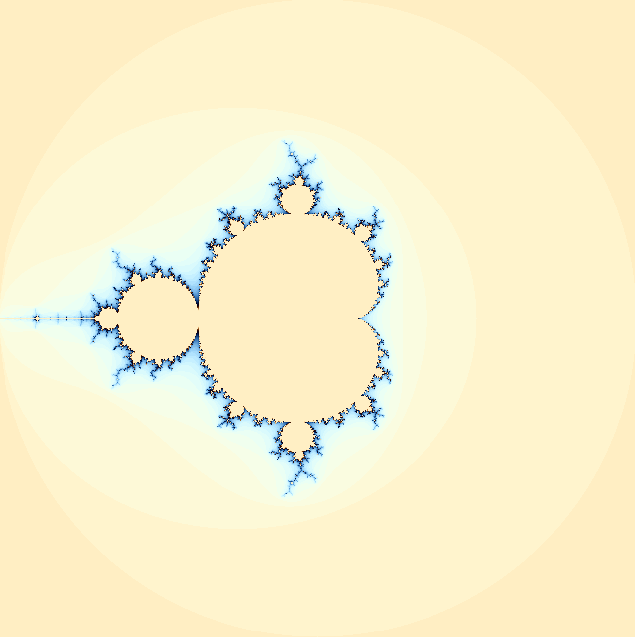A first very interesting property of this program is that the computation for all the points is done only once. It is a bit long before the first image appears, but if you resize the window, it updates instantaneously. This property is a direct consequence of purity. If you look closely, you see that allPoints is a pure list. Therefore, calling allPoints will always render the same result and Haskell is clever enough to use this property. While Haskell doesn’t garbage collect allPoints the result is reused for free. We did not specified this value should be saved for later use. It is saved for us.

See what occurs if we make the window bigger: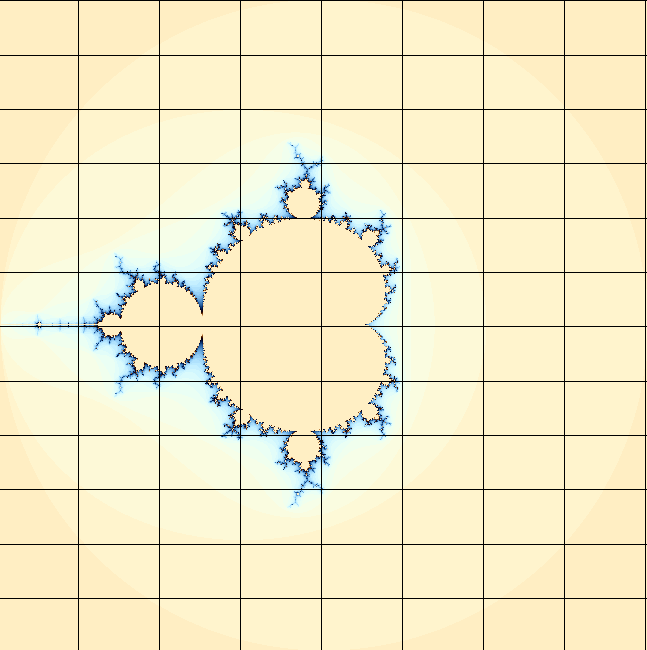We see some black lines because we have drawn less point than there is on the surface. We can repair this by drawing little squares instead of just points. But, instead we will do something a bit different and unusual.

Only the edges

This time, instead of drawing all points, we will simply draw the edges of the Mandelbrot set. The method I use is a rough approximation. I consider the Mandelbrot set to be almost convex. The result will be good enough for the purpose of this tutorial.

We change slightly the drawMandelbrot function. We replace the Points by LineLoop

And now, we should change our list of points. Instead of drawing every point of the visible surface, we will choose only point on the surface.

We only need to compute the positive point. The Mandelbrot set is symmetric relatively to the abscisse axis.

This function is interesting. For those not used to the list monad here is a natural language version of this function:

positivePoints =
for all x in the range [-width..width]
let y be smallest number s.t. mandel x y > 0
if y is on 0 then don't return a point
else return the value corresonding to (x,y,color for (x+iy))

In fact using the list monad you write like if you consider only one element at a time and the computation is done non deterministically. To find the smallest number such that mandel x y > 0 we use a simple dichotomy:

No rocket science here. See the result now: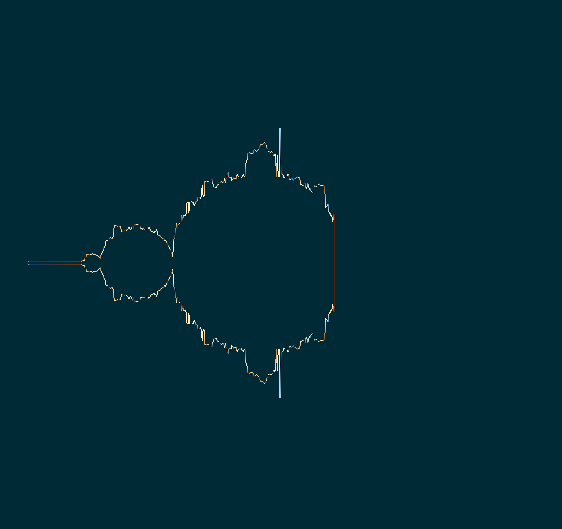3D Mandelbrot?

Now we will we extend to a third dimension. But, there is no 3D equivalent to complex. In fact, the only extension known are quaternions (in 4D). As I know almost nothing about quaternions, I will use some extended complex, instead of using a 3D projection of quaternions. I am pretty sure this construction is not useful for numbers. But it will be enough for us to create something that look nice.

This section is quite long, but don’t be afraid, most of the code is some OpenGL boilerplate. If you just want to skim this section, here is a high level representation:

• OpenGL Boilerplate

• set some IORef (understand variables) for states
• Drawing:

• set doubleBuffer, handle depth, window size…
• Use state to apply some transformations
• Keyboard: hitting some key change the state of IORef

• Generate 3D Object

allPoints :: [ColoredPoint]
allPoints =
for all (x,y), -width<x<width, 0<y<height
Let z be the minimal depth such that
mandel x y z > 0
(x, y, z,color)
(x,-y, z,color)
(x, y,-z,color)
(x,-y,-z,color)
+ neighbors to make triangles

We declare a new type ExtComplex (for extended complex). An extension of complex numbers with a third component:

The most important part is the new multiplication instance. Modifying this formula will change radically the shape of the result. Here is the formula written in a more mathematical notation. I called the third component of these extended complex strange.

real((x, y, z) * (x′, y′, z′)) = xx′ − yy′ − zz

im((x, y, z) * (x′, y′, z′)) = xy′ − yx′ + zz

strange((x, y, z) * (x′, y′, z′)) = xz′ + zx

Note how if z=z'=0 then the multiplication is the same to the complex one.

From 2D to 3D

As we will use some 3D, we add some new directive in the boilerplate. But mainly, we simply state that will use some depth buffer. And also we will listen the keyboard.

The idle is here to change the states. There should never be any modification done in the display function.

We introduce some helper function to manipulate standard IORef. Mainly modVar x f is equivalent to the imperative x:=f(x), modFst (x,y) (+1) is equivalent to (x,y) := (x+1,y) and modSnd (x,y) (+1) is equivalent to (x,y) := (x,y+1)

And we use them to code the function handling keyboard. We will use the keys hjkl to rotate, oi to zoom and sedf to move. Also, hitting space will reset the view. Remember that angle and campos are pairs and zoom is a scalar. Also note (+0.5) is the function \x->x+0.5 and (-0.5) is the number -0.5 (yes I share your pain).

Note display takes some parameters this time. This function if full of boilerplate:

Not much to say about this function. Mainly there are two parts: apply some transformations, draw the object.

The 3D Mandelbrot

We have finished with the OpenGL section, let’s talk about how we generate the 3D points and colors. First, we will set the number of details to 200 pixels in the three dimensions.

This time, instead of just drawing some line or some group of points, we will show triangles. The function allPoints will provide a multiple of three points. Each three successive point representing the coordinate of each vertex of a triangle.

In fact, we will provide six ordered points. These points will be used to draw two triangles.The next function is a bit long. Here is an approximative English version:

forall x from -width to width
forall y from -height to height
forall the neighbors of (x,y)
let z be the smalled depth such that (mandel x y z)>0
let c be the color given by mandel x y z
add the point corresponding to (x,y,z,c)

Also, I added a test to hide points too far from the border. In fact, this function show points close to the surface of the modified mandelbrot set. But not the mandelbrot set itself.

If you look at the function above, you see a lot of common patterns. Haskell is very efficient to make this better. Here is a harder to read but shorter and more generic rewritten function:

If you prefer the first version, then just imagine how hard it will be to change the enumeration of the point from (x,y) to (x,z) for example.

Also, we didn’t searched for negative values. This modified Mandelbrot is no more symmetric relatively to the plan y=0. But it is symmetric relatively to the plan z=0. Then I mirror these values.

The rest of the program is very close to the preceding one.

We simply add a new dimension to the mandel function and change the type signature of f from Complex to ExtComplex.

Here is the result:Naïve code cleaning

The first approach to clean the code is to separate the GLUT/OpenGL part from the computation of the shape. Here is the cleaned version of the preceding section. Most boilerplate was put in external files.

The yMainLoop takes two arguments: the title of the window and a function from time to triangles

We set some global constant (this is generally bad).

We then generate colored points from our function. This is similar to the preceding section.

This code is cleaner but many things doesn’t feel right. First, all the user interaction code is outside our main file. I feel it is okay to hide the detail for the rendering. But I would have preferred to control the user actions.

On the other hand, we continue to handle a lot rendering details. For example, we provide ordered vertices.

Functional organization?

Some points:

1. OpenGL and GLUT is done in C. In particular the mainLoop function is a direct link to the C library (FFI). This function is clearly far from the functional paradigm. Could we make this better? We will have two choices:

• create our own mainLoop function to make it more functional.
• deal with the imperative nature of the GLUT mainLoop function.
As one of the goal of this article is to understand how to deal with existing libraries and particularly the one coming from imperative languages, we will continue to use the mainLoop function.
2. Our main problem come from user interaction. If you ask “the Internet”, about how to deal with user interaction with a functional paradigm, the main answer is to use functional reactive programming (FRP). I won’t use FRP in this article. Instead, I’ll use a simpler while less effective way to deal with user interaction. But The method I’ll use will be as pure and functional as possible.

Here is how I imagine things should go. First, what the main loop should look like if we could make our own:

functionalMainLoop =
Read user inputs and provide a list of actions
Apply all actions to the World
Display one frame
repetere aeternum

Clearly, ideally we should provide only three parameters to this main loop function:

• an initial World state
• a mapping between the user interactions and functions which modify the world
• a function taking two parameters: time and world state and render a new world without user interaction.

Here is a real working code, I’ve hidden most display functions. The YGL, is a kind of framework to display 3D functions. But it can easily be extended to many kind of representation.

We first set the mapping between user input and actions. The type of each couple should be of the form (user input, f) where (in a first time) f:World -> World. It means, the user input will transform the world state.

And of course a type design the World State. The important part is that it is our World State type. We could have used any kind of data type.

The important part to glue our own type to the framework is to make our type an instance of the type class DisplayableWorld. We simply have to provide the definition of some functions.

The camera function will retrieve an object of type Camera which contains most necessary information to set our camera. The objects function will returns a list of objects. Their type is YObject. Note the generation of triangles is no more in this file. Until here we only used declarative pattern.

We also need to set all our transformation functions. These function are used to update the world state.

Note (-*<) is the scalar product (α -*< (x,y,z) = (αx,αy,αz)). Also note we could add two Point3D.

The resize is used to generate the 3D function. As I wanted the time spent to generate a more detailed view to grow linearly I use this not so straightforward formula.

The yMainLoop takes three arguments.

• A map between user Input and world transformation
• A timed world transformation
• An initial world state

Here is our initial world state.

We will define shapeFunc later. Here is the function which transform the world even without user action. Mainly it makes some rotation.

Now the function which will generate points in 3D. The first parameter (res) is the resolution of the vertex generation. More precisely, res is distance between two points on one direction. We need it to “close” our shape.

The type Function3D is Point -> Point -> Maybe Point. Because we consider partial functions (for some (x,y) our function can be undefined).

With the color function.

The rest is similar to the preceding sections.

I won’t explain how the magic occurs here. If you are interested, just read the file YGL.hs. It is commented a lot.

Optimization

Our code architecture feel very clean. All the meaningful code is in our main file and all display details are externalized. If you read the code of YGL.hs, you’ll see I didn’t made everything perfect. For example, I didn’t finished the code of the lights. But I believe it is a good first step and it will be easy to go further. Unfortunately the program of the preceding session is extremely slow. We compute the Mandelbulb for each frame now.

Before our program structure was:

Constant Function -> Constant List of Triangles -> Display

Now we have

Main loop -> World -> Function -> List of Objects -> Atoms -> Display

The World state could change. The compiler can no more optimize the computation for us. We have to manually explain when to redraw the shape.

To optimize we must do some things in a lower level. Mostly the program remains the same, but it will provide the list of atoms directly.

Our initial world state is slightly changed:

The use of eps is a hint to make a better zoom by computing with the right bounds.

We use the YGL.getObject3DFromShapeFunction function directly. This way instead of providing XYFunc, we provide directly a list of Atoms.

We know that resize is the only world change that necessitate to recompute the list of atoms (triangles). Then we update our world state accordingly.

All the rest is exactly the same.

And you can also consider minor changes in the YGL.hs source file.

Conclusion

As we can use imperative style in a functional language, know you can use functional style in imperative languages. This article exposed a way to organize some code in a functional way. I’d like to stress the usage of Haskell made it very simple to achieve this.

Once you are used to pure functional style, it is hard not to see all advantages it offers.

The code in the two last sections is completely pure and functional. Furthermore I don’t use GLfloat, Color3 or any other OpenGL type. If I want to use another library in the future, I would be able to keep all the pure code and simply update the YGL module.

The YGL module can be seen as a “wrapper” around 3D display and user interaction. It is a clean separator between the imperative paradigm and functional paradigm.

If you want to go further, it shouldn’t be hard to add parallelism. This should be easy mainly because most of the visible code is pure. Such an optimization would have been harder by using directly the OpenGL library.

You should also want to make a more precise object. Because, the Mandelbulb is clearly not convex. But a precise rendering might be very long from O(n².log(n)) to O(n³).

1. Unfortunately, I couldn’t make this program to work on my Mac. More precisely, I couldn’t make the DevIL library work on Mac to output the image. Yes I have done a brew install libdevil. But even a minimal program who simply write some jpg didn’t worked. I tried both with Haskell and C.

2. Generally in Haskell you need to declare a lot of import lines. This is something I find annoying. In particular, it should be possible to create a special file, Import.hs which make all the necessary import for you, as you generally need them all. I understand why this is cleaner to force the programmer not to do so, but, each time I do a copy/paste, I feel something is wrong. I believe this concern can be generalized to the lack of namespace in Haskell.

3. I tried Complex Double, Complex Float, this current data type with Double and the actual version Float. For rendering a 1024x1024 Mandelbrot set it takes Complex Double about 6.8s, for Complex Float about 5.1s, for the actual version with Double and Float it takes about 1.6 sec. See these sources for testing yourself: https://gist.github.com/2945043. If you really want to things to go faster, use data Complex = C {-# UNPACK #-} !Float {-# UNPACK #-} !Float. It takes only one second instead of 1.6s.

Published on 2012-06-15
Done with Vim spacemacs & nanoc Hakyll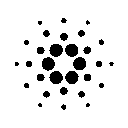ADA: DdzFFzCqrhtAvdkmATx5Fm8NPJViDy85ZBw13p4XcNzVzvQg8e3vWLXq23JQWFxPEXK6Kvhaxxe7oJt4VMYHxpA2vtCFiP8fziohN6Yp